﻿ A Maximin Zero Suffix Method for Quadratic Transportation ProblemAmerican Journal of Operational Research

p-ISSN: 2324-6537    e-ISSN: 2324-6545

2016;  6(3): 55-60

doi:10.5923/j.ajor.20160603.01### A Maximin Zero Suffix Method for Quadratic Transportation Problem

Shambhu Sharma1, Rama Shanker2, Ravi Shanker3

1Department of Mathematics, Dayalbagh Educational Institute, Dayalbagh, Agra, India

2Department of Statistics, Eritrea Institute of Technology, Asmara, Eritrea

3Department of Mathematics, G.L.A. College, N.P. University, Daltonganj, Jharkhand, India

Correspondence to: Rama Shanker, Department of Statistics, Eritrea Institute of Technology, Asmara, Eritrea.
 Email: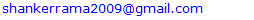Abstract

In the present paper, a maximin zero suffix method introduced by Sharma et al (2014) has been extended to solve the quadratic transportation problem (QTP). The proposed method is better than the existing methods in the literature for solving QTP because it is free from any huge algebraic computation and drawing of graphs of quadratic cost functions to search absolute point. The algorithm of the proposed method has been given and a numerical example has been presented to explain its application. The proposed method is very simple, easy to understand and apply for any practitioners who have not much knowledge of mathematics.

Keywords: Quadratic transportation problem, Estimated cost matrix, Transformed cost matrix, Reduced cost matrix, Zero suffix method, Optimal solution

Cite this paper: Shambhu Sharma, Rama Shanker, Ravi Shanker, A Maximin Zero Suffix Method for Quadratic Transportation Problem, American Journal of Operational Research, Vol. 6 No. 3, 2016, pp. 55-60. doi: 10.5923/j.ajor.20160603.01.

### 1. Introduction

The classical transportation problem (CTP) is a particular class of linear programming problem (LPP) and deals with the distribution of goods (or products) from m sources (plants or suppliers) to n destinations (warehouses or customers). The main objective in CTP is to determine the amount of goods to be shipped from each source to each destination so as to maintain the demand and supply requirements at the minimum total transportation cost. In CTP, the cost of transporting one unit of goods from a source to a destination is independent of the amount of goods shipped.
The classical transportation problem (CTP) is defined as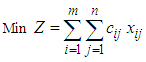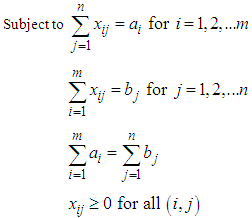where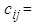Unit transportation cost from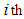source to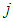th destination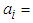Amount of goods available at th source.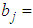Amount of goods required at th destination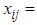Amount of goods transported from th source to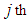destination.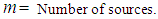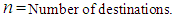CTP, being a well structured problem, has been studied extensively in the literature. It was Hitchcock (1941) who firstly developed the basic transportation problem and suggested constructive method of solution. Later on, Dantzig (1963) formulated the CTP as an LPP and provided the solution. A number of researchers have worked to find suitable methods of solving CTP using different assumptions including Charnes and Cooper (1954), Shih (1987), Arsham and Khan (1989), Adlakha and Kowalski (1999), Ji and Chu (2002), Korukoglu and Balli (2011), Sudhakar et al (2012), are some among others. Recently Sharma et al (2013) have introduced an easy and interesting method named, “A modified zero suffix method” for finding optimal solution for CTP.
In the real life situation, per unit transportation cost of goods does not remain always constant. Generally, it decreases with the increase in the volume of transported goods but not always. Sometimes, it has also been seen that it increases with the increase in the volume of the transported goods due to highway congestion (restriction of over loading). This idea leads to a new dimension of the CTP and generally it has been observed that per unit cost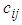is a function of the amount shipped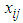. If the per unit transportation costin CTP is a quadratic function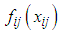of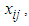then CTP is termed as the quadratic transportation problem (QTP). In QTP, the cost coefficients are quadratic functions, and in CTP the cost coefficients are constants.
Mathematically, the quadratic transportation problem (QTP) is defined as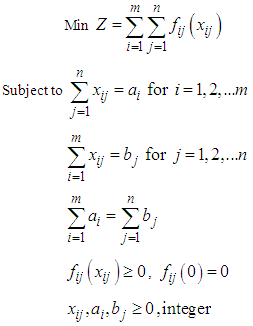There is no any efficient direct algorithm to solve QTP in the literature. However, QTP and its indirect solution techniques have been discussed by researchers including Hochbaum et al (1992), Megiddo and Tamir (1993), Eduardo et al (2003), Cosares and Hochbaum (1994) are some among others. The techniques developed by these researchers are very much complicated and time-consuming. Recently, Adlakha and Kowalski (2013) have introduced an analytical algorithm for QTP which is based on finding absolute point (AP) in QTP by tracing graph for each quadratic cost function which is time-consuming and tedious and needs computer application for tracing graphs of each quadratic cost function.
In the present paper, “A maximin zero suffix method” introduced by Sharma et al (2014) for solving assignment problems has been extended to solve QTP. To use this method, firstly estimated cost matrix of the QTP has to be obtained and then” A maximin zero suffix method” can be applied on the estimated cost matrix. This method is very simple, easy to apply and free from drawing graph of each quadratic cost function. An example of QTP from Adlakha and Kowalski (2013) has been considered to explain the “Maximin zero suffix method” for finding optimal solution.

### 2. Estimated Cost Matrix

Firstly each quadratic cost is estimated to be constant cost. Then idea developed by Sharma et al (2014) is used to solve it. Per unit transporting cost of goods from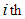source to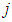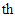destination is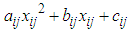where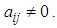If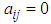and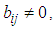the problem is linear transportation problem (LTP). If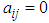and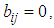the problem is a classical transportation problem (CTP).
The possible allocation to the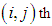cell lies from 0 to min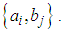The maximum possible allocation to the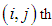cell is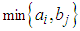and the corresponding cost is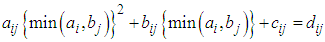(say). The minimum possible allocation cost there is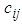for the case of non allocation. Corresponding to the quadratic allocated cost matrix we have an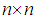estimated cost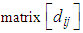. Note that in the classical transportation problem at most cost is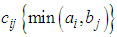and at least cost is 0 in the case of non - allocation.

### 3. A Maximin Zero Suffix Method

Our goal is to find the minimum possible allocation cost which is possible when we allocate the lowest estimated cost cell.
To obtain the minimum allocated cost, we search the least cost cell in the estimated cost matrix for possible allocation. After subtracting the lowest cost of each row from the corresponding row and then subtracting the lowest cost of each column from the corresponding column we get at least one zero in each row and each column in the transformed estimated cost matrix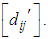The lowest cost cells in each row and each column are detected by these zeros. On minimum cost distribution, zero cells (Lowest cost cells) should be assigned as far as possible satisfying the demand and supply conditions. Our aim is to assign zero cells one by one satisfying corresponding demand and supply conditions in such a manner that over all transporting cost becomes optimal one. The question is to which zero cells should be allocated firstly for getting an optimal transportation schedule.
To overcome this critical situation we focus on two zero cells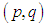and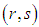of the transformed estimated cost matrix. Let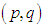cell is assigned and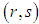cell is not assigned. It means that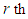supplying source is not used for th demand destination. Then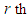supply must be assigned to other demand. To assign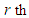supply for minimum cost we select a cell in the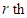row whose cost is the next lowest cost in it’s row. Let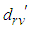is the next minimum in the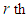row. Then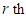supply must be assigned to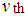demand. So the excess cost in the absence of not assigning to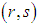cell is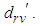Also in the absence of not assigning to the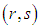cell,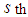demand is not taken by the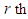supply. To assign a value to the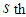demand for minimum cost, we select a cell in the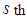column whose cost is the next smallest in its column. Let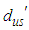is the next minimum in the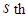column. Then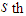demand must be fulfilled by the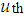supply. So the excess cost in the absence of not assigning to the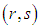cell, the assignment cost may be increased at most by maximum of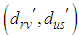= max{lowest cost in its row, lowest cost in its column excluding itself} = suffix value of zero of the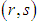cell. Similarly, in case of not assigning to the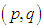cell we may have to face excess cost which is equal to the suffix value of zero of the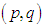cell. Note that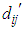is the value ofin the transformed estimated cost matrix. We conclude that to get minimum transportation cost it is better to assign that zero cell whose suffix value is the greatest one.
The methodology of the paper is to block the path of exceeding cost above the optimal one in the process of assigning the zero cells. This is done by finding suffix value of all zeros of the transformed table and assignment is done to the cell having greatest suffix value. Then we have to delete the row/column of assigned cell to get the reduced table. The process is continued in the reduced table till all the demand and supply are exhausted.

### 4. Algorithm

The algorithm of maximin zero suffix method for finding an optimal solution of quadratic transportation problem (QTP) consists of the following steps:
Step 1. Construct the estimated cost matrix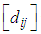where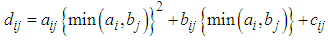Step 2. Subtract the least element of each row from all the elements of the corresponding row of the estimated cost matrix
Step 3. Subtract the least element of each column from all the elements of the corresponding column obtained in step 2 to get the transformed estimated cost matrix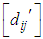Step 4. Find the suffix value of each zero cell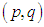using the following formula. Suffix value of the cell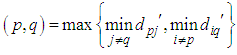Step 5. Assign the cell having the greatest suffix value and delete the exhausted row/column to get the reduced estimated cost matrix
Step 6. In the reduced table update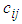for those cells whose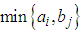is reduced by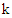as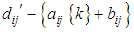Step 7. Repeat steps 2 to 6 till all the demands and supplies are exhausted.

### 5. A Numerical Example

Let us consider the cost matrix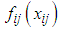for the QTP given in Adlakha and Kowalski (2013).
 Table 1.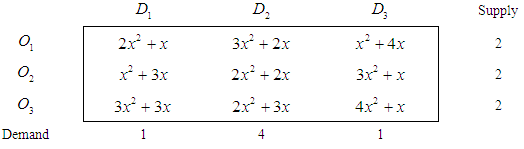Step 1. Estimated cost matrix of the given QTP is
 Table 2.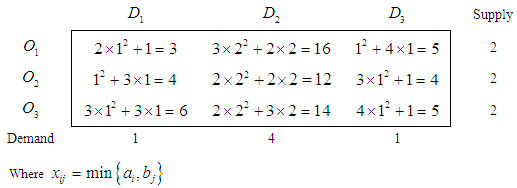Step 2. Subtracting the row minimum from the respective row in table 2, we get
 Table 3.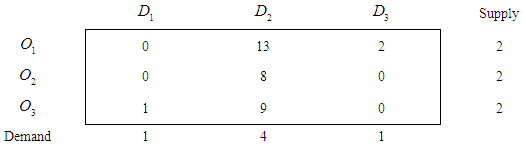Step 3. Subtracting Column minimum from the respective column in table 3, we get
 Table 4.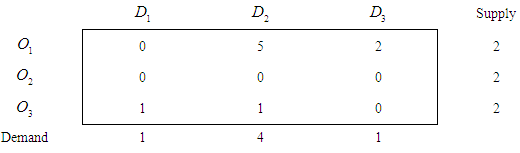Step 4. Suffix Value of each zero in table 4 is shown in table 5 along with the allocated amount to be shipped.
 Table 5.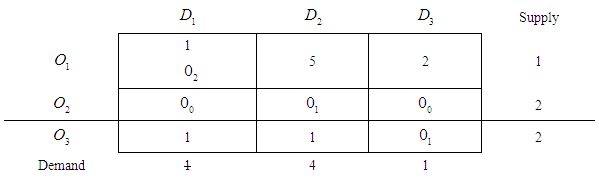Step 5. Reduced estimated cost matrix with updated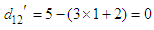has been obtained and shown in table 6 along with suffix value of zeros and allocated amount to be shipped
 Table 6.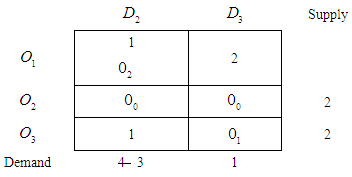Now repeating steps 2 to 6 we get
 Table 7.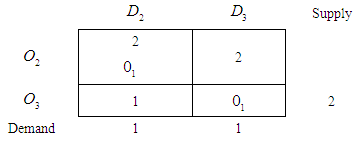Table 8.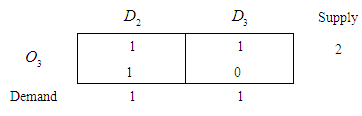Finally an optimal transportation table is as follows: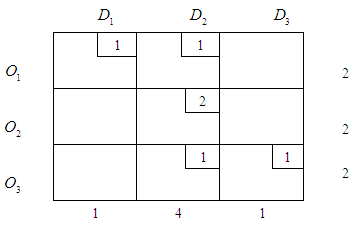The minimum cost for the given QTP can be obtained as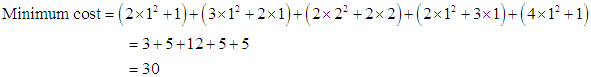It would be recalled that the total minimum transportation cost for the above QTP obtained by Adlakha and Kowalski (2013) is also 30.

### 6. Optimality Criteria and Convergence of the Algorithm

Since we have used the method of minimizing the excess transporting cost corresponding to a cell by assigning the lowest cost cell in each row and each column, there is no chance that the cost obtained according to this process is higher than the optimal cost.
As the number of rows and the number of columns are finite and at each iteration one row or one column is deleted, the algorithm will terminate in a finite number of steps. The number of iterations will be at most m + n and at least max (m, n).

### 7. Concluding Remarks

A maximin zero suffix method has been introduced to solve the quadratic transportation problem (QTP). The proposed method is better than all existing methods in the literature for solving QTP because it is free from any huge algebraic computation and drawing of graphs of quadratic cost functions to search absolute point. The algorithm of the proposed method has been given and the application of the proposed method has been explained by a numerical example. The proposed method is very simple, easy to understand and apply for any practitioners who have not much knowledge of Mathematics and therefore useful for managerial decisions. The proposed maximin zero suffix method for solving QTP can be extended to the polynomial transportation problem (PTP) in which the cost coefficients are higher than second degree polynomials.
The future research on the proposed method is to apply the method for solving real-world problem. For using this method to solve real-world problems a computer program must be developed because the real-world problems consist of several sources and destinations which is difficult to handle manually. Since the proposed method is easy to apply and understand, it can be easily applied to solve real-world problems.

### ACKNOWLEDGEMENTS

The authors would like to thank the Editor-In-Chief and the referee for careful reading and for their constructive comments and suggestions which improved the quality of the paper.

### References

  Adlakha, V. and Kowalski (1999): An Alternative Solution Algorithm for Certain Transportation Problems, International Journal of Mathematical Education in Science and Technology, 30(5), 719 – 728.  Adlakha, V. and Kowalski (2013): On the Quadratic Transportation Problem, Open Journal of Optimization, 2, 89 - 94.  Arsham, H. and Khan, A.B. (1989): A Simplex Type Algorithm for General Transportation Problems – An Alternative to Stepping Stone, Journal of Operational Research Society, 40(6), 581 – 590.  Charnes, A. and Cooper, W.W. (1954): The Stepping Stone Method for explaining Linear Programming Calculations in Transportation Problems, Management Science, 1(1), 49 – 69.  Cosares, S. and Hochbaum, D.S. (1994): Strongly Polynomial Algorithms for the Quadratic Ttransportation Problems with a fixed number of sources, Mathematics of Operations Research, 19(1), 94 – 111.  Dantzig, G.B. (1963): Linear Programming and Extensions, Princeton university press, Princeton, NJ.  Eduardo, M.B., Francisco, S.J.D. and Real, J.R. (2003): Adaptive Productivity Theory to the Quadratic Cost function-An Application to the Spanish Electric Sector, Journal of Productivity Analysis, 20(2), 233 – 249.  Hitchcock, F.L. (1941): The Distribution of a Product from Several Sources to Numerous Localities, Journal of Mathematics and Physics, 20, 224 – 230.  Hochbaum, D.S. Shamir, R. and Shanthikumar, J.G. (1992): A Polynomial Algorithm for an Integer Quadratic Non-Separable Transportation Problem, Mathematical Programming, 55, no 1-3, 359 – 371.  Ji, P. and Chu, K.F. (2002): A Dual Matrix Approach to the Transportation Problem, Asia Pacific Journal of Operations Research, 19 (1), 35 - 45.  Korukoglu, S. and Balli, S. (2011): An Improved Vogel’s Approximation Method for the Transportation Problem, Mathematical and Computational Applications, 16 (2), 370 - 381.  Megiddo, N. and Tamir, A. (1993): Linear Time Algorithms for Some Separable Quadratic Programming Problems, Operations research letters, 13(4), 203 – 211.  Sharma, S., Shanker, R. and Shanker, R. (2013): A Modified Zero Suffix Method for finding Optimal Solution for Transportation Problems, European Journal of Scientific Research, 104(4), 673 – 676.  Sharma, S., Shanker, R. and Shanker, R. (2014): A Maximin Zero Suffix Method for Solving Assignment Problems, European Journal of Scientific Research, 126(2), 206-212.  Shih, W. (1987): Modified Stepping Stone Method as a Teaching aid for Capacitated Transportation Problems, Decision Sciences, 18, 662 - 676.  Sudhakar, V.J., Arunsankar, N. and Karpagam, T. (2012): A new approach for finding an Optimal Solution for Transportation Problems, European Journal of Scientific Research, 68(2), 254 – 257.